# Different ways to represent Signed Integer

• Last Updated : 11 Jan, 2022

A signed integer is an integer with a positive ‘+’ or negative sign ‘-‘ associated with it. Since the computer only understands binary, it is necessary to represent these signed integers in binary form.

In binary, signed Integer can be represented in three ways:

Let us see why 2’s complement is considered to be the best method.

Signed bit Representation

In the signed integer representation method the following rules are followed:

1. The MSB (Most Significant Bit) represents the sign of the Integer.
2. Magnitude is represented by other bits other than MSB i.e. (n-1) bits where n is the no. of bits.
3. If the number is positive, MSB is 0 else 1.
4. The range of signed integer representation of an n-bit number is given as –(2^{n-1}-1) to (2)^{n-1}-1.

Example:

Let n = 4

Range:
–(2^{4-1}-1)    to 2^{4-1}-1
= -(2^{3}-1)    to 2^{3}-1
= -(7)   to+7

For 4 bit representation, minimum value=-7 and maximum value=+7

Signed bit Representation:

Drawbacks:

1. For 0, there are two representations: -0 and +0 which should not be the case as 0 is neither –ve nor +ve.
2. Out of 2^n bits for representation, we are able to utilize only 2^{n-1}  bits.
3. Numbers are not in cyclic order i.e. After the largest number (in this, for example, +7) the next number is not the least number (in this, for example, +0).
4. For negative numbers signed extension does not work.

Example:
Signed extension for +5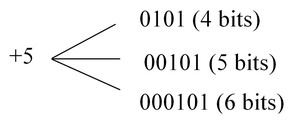Signed extension for -5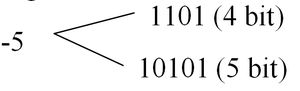5. As we can see above, for +ve representation, if 4 bits are extended to 5 bits there is a need to just append 0 in MSB.
6. But if the same is done in –ve representation we won’t get the same number. i.e. 10101 ≠ 11101.

1’s Complement representation of a signed integer

In 1’s complement representation the following rules are used:

1. For +ve numbers the representation rules are the same as signed integer representation.
2. For –ve numbers, we can follow any one of the two approaches:

• Write the +ve number in binary and take 1’s complement of it.

1’s complement of 0 = 1 and 1’s complement of 1 = 0

Example:
(-5) in 1’s complement:
+5 = 0101
-5 = 1010

• Write Unsigned representation of 2^n-1-X for –X.

Example:
–X = -5      for n=4
2^4-1-5=10 ->1010(Unsigned)

3. The range of 1’s complement integer representation of n-bit number is given as –(2^{n-1}-1) to 2^{n-1}-1.

1’s Complement Representation:

Drawbacks:

1. For 0, there are two representations: -0 and +0 which should not be the case as 0 is neither –ve nor +ve.
2. Out of 2^n bits for representation, we are able to utilize only 2^{n-1} bits.

Merits over Signed bit representation:

1. Numbers are in cyclic order i.e. after the largest number (in this, for example, +7) the next number is the least number (in this, for example, -7).
2. For negative number signed extension works.

Example: Signed extension for +5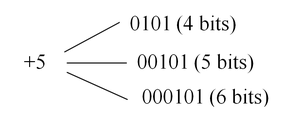Signed extension for -5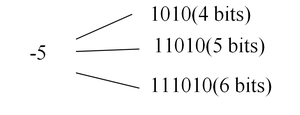3. As it can be seen above, for +ve as well as -ve representation, if 4 bits are extended to 5 bits there is a need to just append 0/1 respectively in MSB.

2’s Complement representation

In 2’s Complement representation the following rules are used:

1. For +ve numbers, the representation rules are the same as signed integer representation.
2. For –ve numbers, there are two different ways we can represent the number.

• Write an unsigned representation of 2^n-X for –X in n-bit representation.

Example:
(-5) in 4-bit representation
2^4-5=11   -→1011(unsigned)

• Write a representation of +X and take 2’s Complement.

To take 2’s complement simply take 1’s complement and add 1 to it.

Example:
(-5) in 2’s complement
(+5) = 0101
1’s complement of (+5) = 1010
Add 1 in 1010:  1010+1 = 1011
Therefore (-5) = 1011

3. Range of representation of n-bit is –(2^{n-1} )  to (2)^{(n-1)-1}.

2’s Complement representation (4 bits)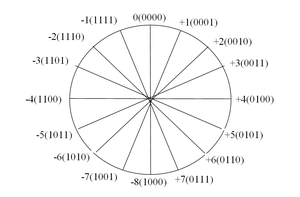Merits:

1. No ambiguity in the representation of 0.
2. Numbers are in cyclic order i.e. after +7 comes -8.
3. Signed Extension works.
4. The range of numbers that can be represented using 2’s complement is very high.

Due to all of the above merits of 2’s complement representation of a signed integer, binary numbers are represented using 2’s complement method instead of signed bit and 1’s complement.

My Personal Notes arrow_drop_up
Recommended Articles
Page :